Skip to main content

# Performance analysis of multi-rate signal processing digital filters on FPGA

## Abstract

Multi-rate signal processing, an important part of the design of a digital frequency converter, is realized mainly based on interpolation and decimation, which match the sampling rate between the baseband and high-frequency processing side, especially in down conversion. However, the design of a digital filter is important for realizing multi-rate interpolation and decimation, which is highlighted in this paper. To analyze the digital filter performance in multi-rate signal processing, the ordinary finite impulse response (FIR) filter and more efficient digital filter are discussed respectively. The ordinary FIR filters use a Hamming window to design, while a more efficient digital filter includes a cascaded integrate comb (CIC) and half-band filter. Sampling rate transformation factor is 12 in this design, which is cascaded by three stages. Each stage corresponding to the conversion factor is 3, 2, and 2. Both of these design methods are implemented on the FPGA development board. The hardware resource occupancy and the error rate of the signal amplitude in decimation show that the efficient digital filter is superior to the digital filter designed by the Hamming window in the real-time processing.

## Introduction

Multi-rate signal processing is the key technology to realize the digital frequency converter. In a general communication system, the rate of the baseband signal is often much lower than that of the intermediate frequency (IF) signal; in order to match the sampling rate of both sides, there is no doubt that the sampling rate of the former increases, which is equivalent to the increase in the number of sampling points. While in the reception process, signals from an analog to digital converter (ADC) with a higher rate are difficult to provide directly to processors for processing; as a result, extraction is considered to decrease the sampling rate. In other words, the discrete sampled signal is resampled, and ultimately, the signal frequency will be down to the appropriate point for data recovery. So interpolation and extraction is not only the basis of multi-rate signal processing, but also an important theoretical support for digital inverter design .

As the functional requirements of the system become more and more diversified, the function of the circuit module in the design becomes more and more complicated, and the higher processing speed of the chip is of great need. In other words, traditional analog circuits cannot meet the whole design demands. So those large-scale integrated devices, such as FPGA and DSP as high-speed signal processors, have been vigorously developed and promoted, which is characterized by the use of digital or software to replace the analog circuit . At present, most of the transceivers include baseband signal processing, digital frequency conversion, and analog conversion. Analog frequency conversion aims at moving high-frequency analog signal to the appropriate IF, which can reduce the restrictions of key devices such as AD/DA, and digital frequency conversion is prone to get a lower rate baseband signal .

This paper is divided into four parts: Section 1 tells about multi-rate signal processing in the digital frequency conversion design and further introduces its development status quo; finally, article structure is also explained. Section 2 mentions two different digital filter designs in multi-rate signal processing, including ordinary FIR filter and CIC, half-band filter as more efficient digital filters, and provides their design schemes. Section 3 gives the detailed implementation progress and simulation results of these two digital filter designs in FPGA. Section 4 is about the conclusion of this paper. Digital down conversion is designed as an example in this paper; decimation will be discussed in the later chapters. When sampling conversion factor is somewhat large, a single-stage filter would need more filter orders than multi-stages, so a three-stage filter cascade in this design is to complete the sampling rate conversion factor of 12, and each stage of decimation factor is 3, 2, and 2. The whole design block diagram is shown in Fig. 1.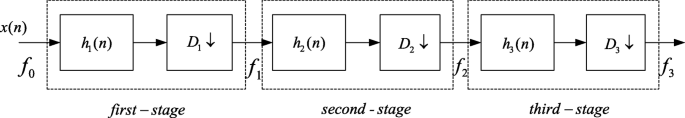Fig. 1

## Methods

The simulations are based on FPGA Altera Develop Board. All the simulation results are calculated by ModelSim and Matlab2018.

## Two designs of digital filter in multi-rate signal processing

Multi-rate signal processing is an important technique to be discussed. It consists of interpolation and decimation and also the corresponding design of digital filter before extraction or after interpolation. The composition of a digital frequency converter is similar to that of the analog frequency converter, mainly including the digital mixer, numerical control oscillator (NCO), and the low-pass filter. In comparison, analog frequency converter’s circuit functions are not stable enough and greatly affected by the temperature. However, a digital frequency converter not only can guarantee the orthogonality of IF carrier, but also conveniently modify the frequency interval and other parameters. Besides, the purpose of the sampling rate conversion is to match the rate of data processing, especially in receiver design; the frequency of the received IF signal after certain decimation will be reduced, thereby relieving the stress of subsequent data processing.

Assuming that an input signal is x(n), the sampling rate is F = 1/T, and the input signal is full of the whole frequency, that is, −F/2 ≤ f ≤ F/2, the digital angular frequency ω = 2πfT. The output of sampling rate conversion is y(n), and the sampling rate changes to F' = 1/T'. The process of sampling rate conversion block diagram is shown in Fig. 2.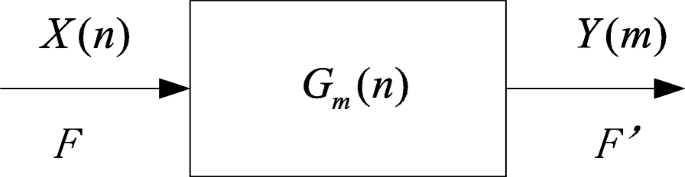Fig. 2

So the input and output sampling rate conversion ratio we can get is:

$$F/{F}^{\hbox{'}}={T}^{\hbox{'}}/T=D/L$$
(1)

where D and L are positive integers, since it is a linear system, and the output sample sequence is also a linear combination of input samples, which can be expressed by Eq. (2):

$$y(m)=\sum \limits_{n=-\infty}^{+\infty }{g}_m(n)\cdot x\left(\left\lfloor m\cdot D/L\right\rfloor -n\right)$$
(2)

when D = L = 1, it becomes a normal impulse response of the linear system in the case of constant sampling rate as we know.

From Eq. (1), when F > F', the sampling rate gets lower, called decimation, according to the Fourier transform; it can be seen that the decimation of the signal in the time domain is equivalent to the broadening of the frequency domain. WhileF < F', the sampling rate is rising up, called interpolation, which leads to the compression of the signal spectrum. Based on that theory, it can be known that the addition of the appropriate threshold filter before decimation or after interpolation can keep the spectral characteristic of the original signal. In addition, when the ratio in Eq. (1) is not a positive integer, then that is a fractional transform, which is achieved by L-times interpolation first and then the D-fold decimation; the structure is shown in Fig. 3, the L-times interpolation is on the left of the block diagram and the D-decimation is on the right.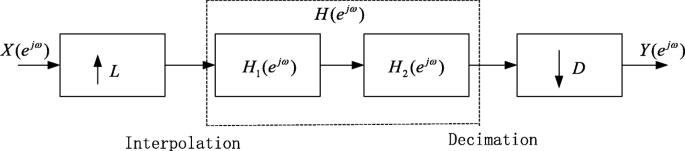Fig. 3

From the above description, it can be seen that the realization of interpolation and decimation depends on the filter design. The filter is designed to meet the input and output amplitude stability and anti-spectral aliasing; what is more, filter efficiency is also an important factor needed to be considered, because it affects real-time signal processing [4, 5]. Two different digital filter designs will be discussed in the following part, the ordinary digital filter using a Hamming window FIR for design will be described first, and then the more efficient digital filter, including the CIC, half-band, and CIC compensation filter will be discussed after.

### Hamming window FIR filter for decimation design

From Fig. 1, it can be known that the sampling rate of each stage after filtered decimation is fk, which can be calculated by Eq. (3):

$${f}_k=\frac{f_{k-1}}{D_k},k=1,2,3$$
(3)

The input signal passband cutoff frequency fp is set to 2.1 MHz in the system simulation, and in order to prevent the spectral aliasing caused by the spectrum extension after decimation, the passband and transition zone of the last stage are designed as follows:

$$\left\{\begin{array}{c}0\le f\le {f}_p=2.1\mathrm{MHz},\kern1em \\ {}{f}_p\le f\le {f}_3/2=2.5\mathrm{MHz}\end{array}\right.$$
(4)

The methods to get passband and transition of the following second- and first-stage filters are designed in such a way that the passband of each stage are the same, while the steepness of transition zone directly affects the order of the filter design. In line with the anti-aliasing filter design, the second transition zone can be calculated.

$${f}_p\le f\le {f}_2-{f}_3/2=7.5\mathrm{MHz}$$
(5)

Similarly, the transition of first stage is

$${f}_p\le f\le {f}_1-{f}_3/2=17.5\mathrm{MHz}$$
(6)

The above design gives the passband, stopband, and the output sampling rate of each stage. A filter out-of-band attenuation of not less than 55 dB is required; from these, the filter order can be calculated by the characteristic of the Hamming window. The specific formula is presented as follows:

$${\mathrm{num}}_i=\left\lceil 6.6\pi \frac{f_{i-1}/2}{f_{\mathrm{stop}}-{f}_p}\right\rceil, i=1,2,3$$
(7)

Respectively, fp and fstop are the passband and stopband cutoff frequency for each stage. Therefore, calculating the order required for each stage of the filter, there will be more efficient digital filter design for decimation.

It can be seen that the third stage of the Hamming window digital filter whose order is up to 261, results in heavy calculations and poor real time. Therefore, a more efficient digital filter is taken into consideration. The CIC and half-band filter as more efficient filters are more common in multi-rate processing, of which the characteristics will be introduced in the following parts.

### The more efficient digital filters

#### CIC filter

CIC known as cascaded integrate comb is one kind of commonly used high efficiency filters, mainly applied when the decimation factor is not the power of 2. The reason for its high efficiency is that its coefficients are 1, so it requires no multiplier. Because of this, it is often used in multi-rate transformations of high-speed signal processing.

The impulse response of the CIC filter is as follows:

$$h(n)=\left\{\begin{array}{c}1,0\le n\le D-1\\ {}0,\kern1.25em \mathrm{other}\kern1.5em \end{array}\right.$$
(8)

where D is the decimation factor, and also representing the order of CIC, the transfer function on the Z-plane is:

$$H(z)=\frac{1}{1-{z}^{-1}}\cdot \left(1-{z}^{-D}\right)$$
(9)

Eq. (9) can be seen as a two-part cascade by the sign of multiplication; the former is an integrator and the latter is a comb, which is the origin of the CIC name. The block diagram is shown in Fig. 4.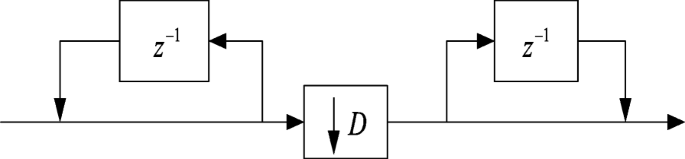Fig. 4

Upon substitution of z = e into Eq. (9), we get the final amplitude frequency response:

$$H\left({e}^{j\omega}\right)=\frac{\sin \left(\omega D/2\right)}{\sin \left(\omega /2\right)}$$
(10)

From this, the amplitude frequency curve of the CIC filter can be obtained (Fig. 5).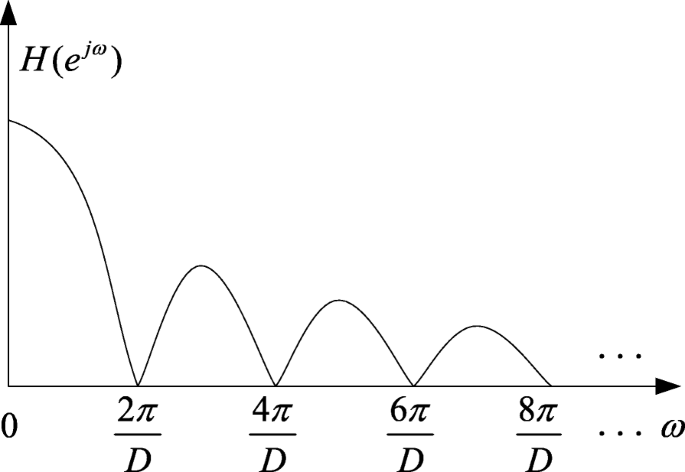Fig. 5

when D >  > 1, the first side lobe amplitude is:

$$A={\left|H\left({e}^{j\omega}\right)\right|}_{\omega =\frac{3\pi }{D}}=\frac{1}{\left|\sin \left(\frac{3\pi }{2D}\right)\right|}\approx \frac{2D}{3\pi }$$
(11)

From Eq. (11), we can know the ratio of the main and side lobe amplitude is 3π/2; that is, the side lobe is only less than the main lobe with 13.46 dB. Therefore, the single CIC filter has a large side lobe amplitude, which generally cannot meet the system requirements. In order to reduce side lobe attenuation, a multi-stage CIC filter cascade is used to aid the design; for each additional stage of the CIC filter, side lobe attenuation will be reduced by 13.46 dB. In this design, five CIC filter cascades make the first side lobe attenuation up to 67 dB, which can basically meet the system requirements [6, 7].

#### Half-band filter

Figure 6 shows the amplitude frequency curve of the half-band filter. In Fig. 6, δp and δs are passband ripple and stopband ripple, respectively, and ωp and ωA are passband cutoff frequency and stopband cutoff frequency. The half-band filter has the following properties:

$${\omega}_p=\pi -{\omega}_A$$
(12)
$${\delta}_p={\delta}_s$$
(13)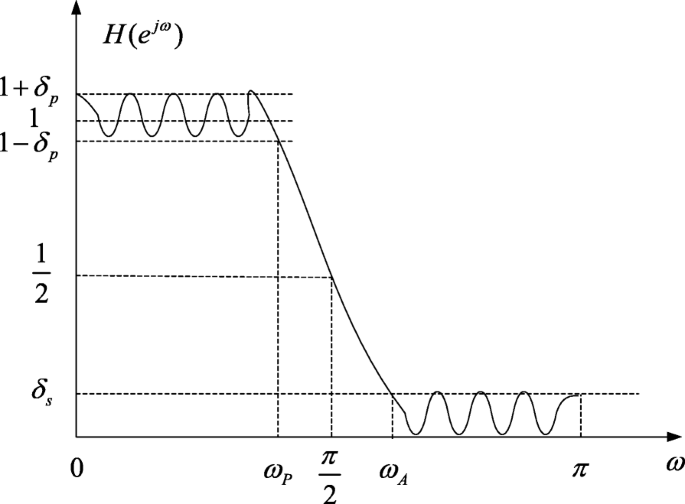Fig. 6

In conjunction with the spectral characteristics of the half-band filter, it can be seen that when δp = δs is small, as for a twofold decimation, the spectrum is twice stretched and then the 2π phase is shifted. Although the spectrum ranging fromπ/2 to ωA is aliased to the signal in [ωp, π/2], the signal located in passband [0, ωp] can be recovered, so that the half-band filter can be used for twofold decimation. When the decimation factor is large and exactly the same as 2M, an M-stage half-band filter cascade can be implemented .

The half-band filter ensures phase linearity and the coefficients of the filter are in even symmetry, which makes it possible to prove that all even coefficients except the center point coefficients are zero, which greatly reduces the amount of filter calculation and increases the real-time filter processing.

#### CIC compensation filter

The received signal can be recovered after being processed by the digital frequency converter, which needs an appropriate design of filter parameters so that the signal waveform shape does not change significantly, but also needs to keep the amplitude of the signal within the fault tolerance range; therefore, the input and output gain remains at 0 dB.

The CIC filter is different from the general FIR filter; it can be seen from the curve of Fig. 5 that the passband is very narrow and not flat; as a result, it cannot be used directly in many applications, so it is necessary to design a corresponding compensation filter so that the CIC filter is flat in the pass band. The N-stage CIC filter cascade frequency response function can be expressed as follows (differential delay M is set to 1 as default):

$$\left|H(f)\right|={\left|\frac{\sin \left(\pi f\right)}{\sin \left(\pi f/R\right)}\right|}^N$$
(14)

where f represents the sampling rate before decimation, R is the sampling rate transform factor, and N is donated as the series of the CIC filter. The larger the parameter N, the more uneven the passband is, so an inverse frequency response in the passband is needed to correct this unevenness [9,10,11]. Thus, the response function of the CIC compensation filter can be deduced according to Eq. (14).

$${H}_{\mathrm{comp}}(f)={\left|R\left(\frac{\sin \left(\pi f/R\right)}{\sin \pi f}\right)\right|}^N\overset{R\to \infty }{\approx }{\left|\frac{\pi f}{\sin \left(\pi f\right)}\right|}^N\kern0.5em ={\left|\sin {c}^{-1}(f)\right|}^N$$
(15)

From Eq. (15), when R → ∞, the compensation filter can be approximated as an inverse Singer function.

#### Design scheme

Digital down conversion is designed as an example in this experiment, assuming that the demodulated signal is a sinusoidal two-tone signal; their frequencies are 1 MHz and 1.5 MHz. The demodulated digital sampling rate is 60 MHz, while the processing rate of back-end DSP is 5 MHz, so the sampling rate conversion factor is 12. A three-stage decimation cascade is designed to complete the down-conversion multi-rate processing. The CIC filter is put on the first stage due to its suitability for high-speed processing, which achieves threefold sampling rate conversion; the second stage is the CIC compensation filter, which is used to reduce the non-linear effects after the CIC filter, and the factor is 2; the last stage is with a half-band filter to have two times sampling rate reduction; the whole down-conversion process is shown in Fig. 7.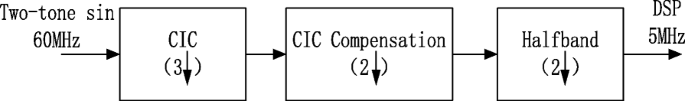Fig. 7

The compensation filter is designed by using Matlab fir2 function, and the coefficient is represented by an 18-bit fixed point; simultaneously, the passband cutoff frequency is set to 2.1 MHz, and the filter order is calculated as 61. Figure 8 shows the CIC filter, CIC compensation filter, and the whole frequency response.; it can be seen that the frequency response of the CIC is not flat in the passband before the compensation, and after the appropriate compensation filter design, the whole response is fairly stable so that the results of filter output do not appear to have too much fluctuations after the proper data bit truncation.Fig. 8

Similarly, the coefficient of the half-band filter is generated using Matlab function “firhalfband” and also represented by an 18-bit fixed point.

## Implementation of two multi-rate digital filter designs

### Implementation of the Hamming window digital filter on FPGA

According to the system requirements, the filter passband cutoff frequency is 2.1 MHz, and the out-of-band attenuation is less than 50 dB. So the characteristic curve of each stage filter is shown in Fig. 9; the passband, stopband cutoff frequency, and sampling rate for each stage of the filter are given in the graph. On the basis of the formula of the Hamming window filter given in Section 2, the filter order of each stage can be calculated as 42, 40, and 261 respectively. It can be seen that the transition band of the first-stage decimation filter is relatively narrow, so the required filter order is large, and the storage resources is also very large for the need, which greatly affects the filter processing speed. Therefore, the parameters of each filter before the decimation are shown in Table 1.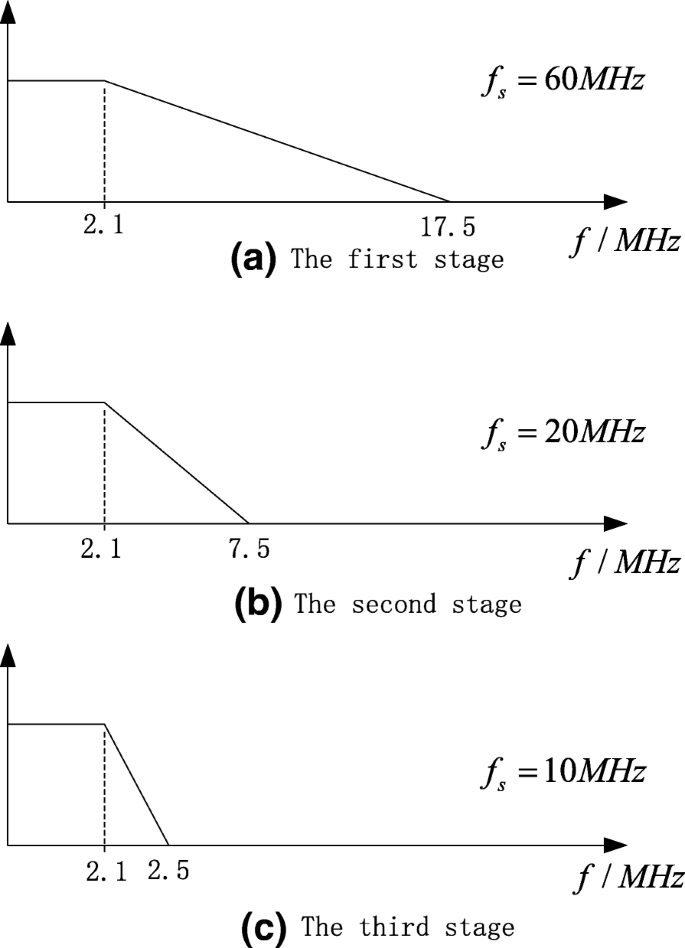Fig. 9

According to the above design of the Hamming window filter, in the hardware simulation, the input is written into the incentive test file by the way of the test input, and the output results are simulated by ModelSim. There are 240 two’s complement data values representing the two-tone signal as the test input; the output is also the two’s complement signal after filtering. The waveform of input and output is basically the same by the simulation tool, and the peak-to-peak amplitude is also close.

### Implementation of the more efficient filter on FPGA

The CIC filter requires only a small amount of adders and registers to implement the decimation process without the need for additional multiplier calculations. The CIC filter consists of an integrator and a differentiator; a single-stage CIC has small side-lobe attenuation and low signal to noise ratio, so a five-CIC filter cascade has been designed in this test. The block diagram of the integrator and the differential are shown in Fig. 10a and b, and the five-cascade decimation of the block diagram is also shown in Fig. 10c below.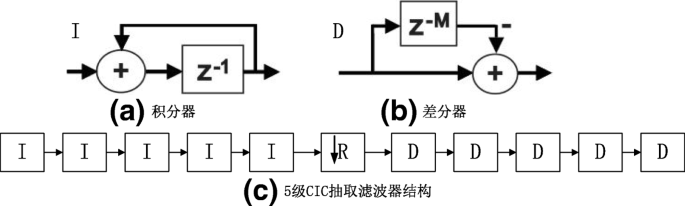Fig. 10

The specific parameters of CIC filter is set as below (Table 2).

The bit of input signal is 16 bits, and the output bit is also set to 16 bits, but the full resolution output will inevitably exceed the effective data bits, so the final results will be generated by the decision of low-bit truncation. In the design, the CIC parameter is set, including conversion factor R = 3, cascade stage N = 5, and differential delay M = 1. The bit width of the CIC filter output is related to the filter gain; for the decimation filter, the output gain is as follows.

$$G={(RM)}^N$$
(16)

Therefore, the full resolution output bit width is:

$${B}_{\mathrm{out}}=\left\lceil {B}_{\mathrm{in}}+N{\log}_2(RM)\right\rceil$$
(17)

By the calculation, a CIC decimation filter gain in this design of 243 can be obtained, that is 23.85 dB. The full-resolution output bit width is 24 bits; in order to get the result with the bit width of 16 bits, the least 8 bits should be truncated. Although some error exists in this processing, fortunately, the error can be calculated by analysis [12, 13].

The input signal uses a two-tone signal with frequencies of 1 MHz and 1.5 MHz, of which the amplitude is normalized. To prevent the amplitude calculation from overflowing in the filter processing, the normalized amplitude is multiplied by 0.2, then the value is stored in 16-bit two’s complement. The corresponding analog format output is shown in Fig. 11. As can be seen from the results, after the CIC filter, the waveform did not change much, but the resolution decreased; this is because the sampling rate is reduced by three times. What is more, the CIC filter gain and output truncation produce a certain error to the signal amplitude, but the error can be calculated by 243/256 = 0.9492, which is consistent with the simulation results.Fig. 11

The second-stage filter is the compensation filter, which mainly compensates the error caused by the non-linear effects of the CIC filter. Figure 12 shows the results of the compensation filter. It can be seen that some part of the waveform has been compensated as marked. The filter coefficients are also expressed by the 18-bit fixed point, which is equivalent to amplifying the signal by 217; one bit represents the sign, and the upper 16 bits of the MSBs are intercepted and the low 19 bits of data is discarded (the maximum coefficient of the compensation filter is greater than 1, so 19 bits are used to store in the hardware actually).Fig. 12

The half-band filter as the last stage whose passband cutoff frequency is set to 2.1 MHz; the ripple factor is 0.0001, using the minimum order of the design method to get the filter order of 59. Its coefficients are also represented by 18-bit fixed points, and the way of output truncation is similar to that of the second-stage filter. The half-band FIR device specification in Altera cyclone IV is displayed in Fig. 13.Fig. 13

The simulation results of the half-band filter are shown in Fig. 14, and it can be seen that the signal characteristics in the passband are not lost.Fig. 14

The waveform characteristics of the signal after filtering can be preserved, but the amplitude of the signal will change. Fortunately, the amplitude error of each filter can be roughly estimated, so adding a simple multiplier at the last stage can compensate for the whole filter output. Using the same simulation method to test the Hamming window digital filter, compared to the more efficient digital filter in the calculation of time, has been greatly improved. The output delay of the Hamming window filter is 17.547 μs, while the more efficient filter is 4.550 μs, simulated on the same computer, with the processor Intel i3 Core, clock 2.4 GHz.

There is a 12-time ratio between the filter input and output sampling points; the way of conventional variance to determine the amplitude fluctuation after decimation is inappropriate, so the peak-to-peak value of the input and output is used to measure this error η, which can be calculated as follows.

$$\eta =\frac{\left|{v}_{\mathrm{in}}-{v}_{\mathrm{out}}\right|}{v_{\mathrm{in}}}$$
(18)

where vin and vout are the difference of the maximum and minimum of the signal amplitude. From the simulation results of the design methods of two different digital filters, the amplitude range of the input signal is [− 6553, 6553] and the output range after decimation by the Hamming window filter is [− 4931, 5404], while the more efficient filter output range is [− 5463, 5923], so the amplitude errors are calculated, η1 = 0.211 and η2 = 0.131, respectively.

The results of the two digital filter design methods are compared with the input and output delay of the filter; the hardware resource occupancy and the amplitude error of the recovery signal. The details are shown in Table 3.

## Results and discussion

In this experiment, the digital filter is designed in multi-rate signal processing in digital down-conversion process and verified its performance on FPGA. Compared with conventional digital filter, the more efficient filter has a great advantage on the real time and the use of hardware resources, which can improve the real-time performance of the signal processing and greatly reduce the rate of the back-end digital signal processing. It is convenient and requires relatively small resources to implementation, which has great application prospect.

## Abbreviations

ADC:

Analog to digital converter

CIC:

Cascaded integrate comb

IF:

Intermediate frequency

NCO:

Numerical control oscillator

## References

1. 1.

B. Sklar, Digital communications: Fundamentals and applications, 2nd edn. (Prentice Hall, California, 2017), pp. 177–180

2. 2.

T.G. Hodgkinson, Wireless communications — The fundamentals. BT Technol. J. 25(2), 11–26 (2007)

3. 3.

M. Cummings, S. Haruyama, FPGA in the software radio. IEEE Commun. Mag. 37(2), 108–112 (1999)

4. 4.

J. Candy, G. Temes, Interpolation and Decimation of Digital Signals: A Tutorial Review Proceeding of the IEEE. 69(3), 300–330 (1981)

5. 5.

R.E. Crochiere, L. Rabiner, Interpolation and decimation of digital signals—A tutorial review. Proc. IEEE 69(3), 300–331 (1981)

6. 6.

W. Feng, CIC filter design and analysis in multiple sampling. Mod. Electron. Technol. 30(14), 18–20 (2007)

7. 7.

X. Liu, Y. Han, G. Liang, et al., in Electron devices and solid-state circuits. Design and implementation of a modified high performance and low power CIC interpolation filter. https://doi.org/10.1109/EDSSC.2011.6117685

8. 8.

Y. Liu, Q. Lin, Half-band filter principle and design—An decimation filter with 2:1 decimation factor. PI 1, 36–40 (1995)

9. 9.

E. Hogenauer, An economical class of digital filters for decimation and interpolation. IEEE Trans. Acoust. Speech Signal Process. 29(2), 155–162 (1981)

10. 10.

J. Zheng, L. Ge, B. Li, Modification of CIC decimation filter and its FPGA realization. J. Inf. Eng. Univ. 7(1), 57–59 (2006)

11. 11.

A.Y. Kwentus, Z. Jiang, A.N. Willson, Application of filter sharpening to cascaded integrator-comb decimation filters. IEEE Trans. Signal Process. 45(2), 457–467 (1997)

12. 12.

Altera Corporation. CIC MegaCore Function User Guide V. 11.0, 2011

13. 13.

Altera Corporation. FIR Compiler User Guide, V. 11.0,2011

Download references

### Funding

This research was supported by “Fundation of Graduate Innovation Center in Nanjing University of Aeronautics and Astronautics (KFJJ20181502)”.

### Availability of data and materials

Data sharing not applicable to this article as no datasets were generated or analyzed during the current study.

## Author information

Authors

### Contributions

YL carried out the simulation and JT carried out the calculation study. QJ participated in the design of the whole experiments. All authors read and approved the final manuscript.

### Corresponding author

Correspondence to Qingfeng Jing.

## Ethics declarations

### Competing interests

The authors declare that they have no competing interests.

### Publisher’s Note

Springer Nature remains neutral with regard to jurisdictional claims in published maps and institutional affiliations.

## Rights and permissions

Open Access This article is distributed under the terms of the Creative Commons Attribution 4.0 International License (http://creativecommons.org/licenses/by/4.0/), which permits unrestricted use, distribution, and reproduction in any medium, provided you give appropriate credit to the original author(s) and the source, provide a link to the Creative Commons license, and indicate if changes were made.

Reprints and Permissions